# 12-267/Homework Assignment 4

This assignment is due at the tutorial on Tuesday October 16. Here and everywhere, neatness counts!! You may be brilliant and you may mean just the right things, but if your readers have a hard time deciphering your work they will give up and assume it is wrong.

Task 0. Identify yourself in the Class Photo!

Task 1. Find the general solution of the Euler-Lagrange equation corresponding to the functional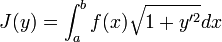$J(y)=\int_a^bf(x)\sqrt{1+y'^2}dx$, and investigate the special cases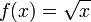$f(x)=\sqrt{x}$ and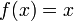$f(x)=x$.

Task 2. Find the extrema the following functional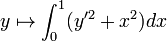$y\mapsto\int_0^1(y'^2+x^2)dx$ subject to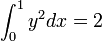$\int_0^1y^2dx=2$ and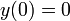$y(0)=0$ and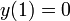$y(1)=0$. (An earlier version of this assignment had by mistake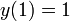$y(1)=1$, which leads to much uglier numbers. If you already solved the problem with$y(1)=1$, you may submit either solution).

Task 3. Solve the "power line problem": Of all the curves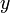$y$ with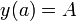$y(a)=A$ and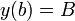$y(b)=B$ and with total arc-length$l$, find the one with the least potential energy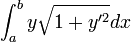$\int_a^by\sqrt{1+y'^2}dx$.

Task 4. Find a necessary condition for a function$y$ satisfying$y(a)=A$,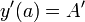$y'(a)=A'$,$y(b)=B$, and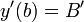$y'(b)=B'$ to be an extremal of a functional of the form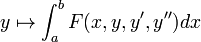$y\mapsto\int_a^bF(x,y,y',y'')dx$.

Task 5. Find the curve$y$ joining the points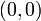$(0,0)$ and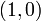$(1,0)$ and for which the integral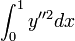$\int_0^1y''^2dx$ is minimal, if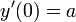$y'(0)=a$ and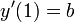$y'(1)=b$.

 Dror's notes above / Student's notes below

Solution to Task 4. --Twine 17:54, 24 October 2012 (EDT)

Let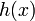$h(x)$ be any function defined on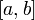$[a, b]$ with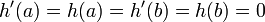$h'(a) = h(a) = h'(b) = h(b) = 0$. For y to be an extremal of the functional with the boundary constraints given, we must have that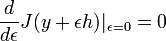$\frac{d}{d\epsilon} J(y + \epsilon h) |_{\epsilon = 0} = 0$ for any such$h(x)$.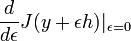$\frac{d}{d\epsilon} J(y + \epsilon h) |_{\epsilon = 0}$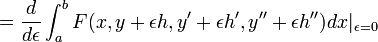$= \frac{d}{d\epsilon} \int_a^b F(x, y+\epsilon h, y'+\epsilon h', y'' +\epsilon h'')dx|_{\epsilon = 0}$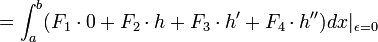$= \int_a^b (F_1 \cdot 0 + F_2 \cdot h + F_3 \cdot h' + F_4 \cdot h'')dx|_{\epsilon = 0}$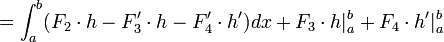$= \int_a^b (F_2 \cdot h - F_3' \cdot h - F_4' \cdot h')dx + F_3 \cdot h|_a^b + F_4\cdot h'|_a^b$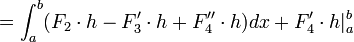$= \int_a^b (F_2 \cdot h - F_3' \cdot h + F_4'' \cdot h)dx + F_4'\cdot h|_a^b$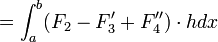$= \int_a^b (F_2 - F_3' + F_4'') \cdot h dx$

For this to be equivalent to 0 for any h defined above, we must have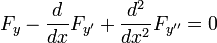$F_y - \frac{d}{dx}F_{y'} + \frac{d^2}{dx^2}F_{y''} = 0$

Solution to Task 5. --Twine 17:54, 24 October 2012 (EDT)

We use the result of 4. As in this case F is independent of x, y, and y', the equation reduces to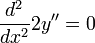$\frac{d^2}{dx^2}2y'' = 0$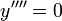$y'''' = 0$

This has the solution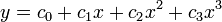$y = c_0 + c_1 x + c_2 x^2 + c_3 x^3$. We can use the constraint equations$y(0) = 0$,$y(1) = 0$,$y'(0) = a$,$y'(1) = b$ to show that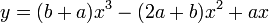$y = (b+a)x^3 - (2a + b)x^2 + ax$. Hence, This is the only y for which the functional is extremal.